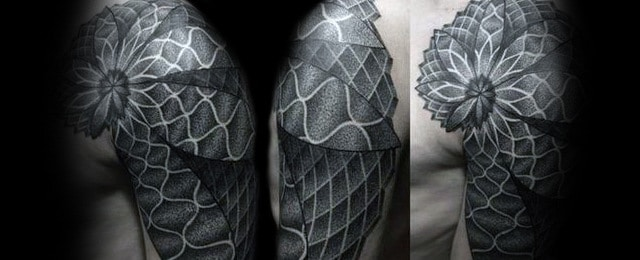# 60 Fibonacci Tattoo Designs For Men – Spiral Ink Ideas

The Fibonacci sequence is a sequence of numbers in which each number is the sum of the two previous numbers. Starting from zero, it goes 0, 1, 1, 2, 3, 5, 8… and so on forever.

The sequence is famous because it appears in many important works of art and architecture as well as in nature.

For example, the shell of a snail and of other animals takes the form of a spiral, and it is possible to draw out this same spiral using the Fibonacci sequence and some geometry. Although it appears to be a random assortment of numbers, the sequence is a symbol of the power of mathematics and order in a world that at first glance seems chaotic.

You can wear a Fibonacci tattoo in a number of ways. For example, you can look up a spiral that obeys the Fibonacci rule for its design and use that. It can be of almost any size because it is composed of simple geometric lines. You can also get a rectangular design that works the same way if you prefer that.

Either way, it will be a signal of intelligence and insight into the laws that govern how the physical law operates.

## 1. Forearm Fibonacci Tattoos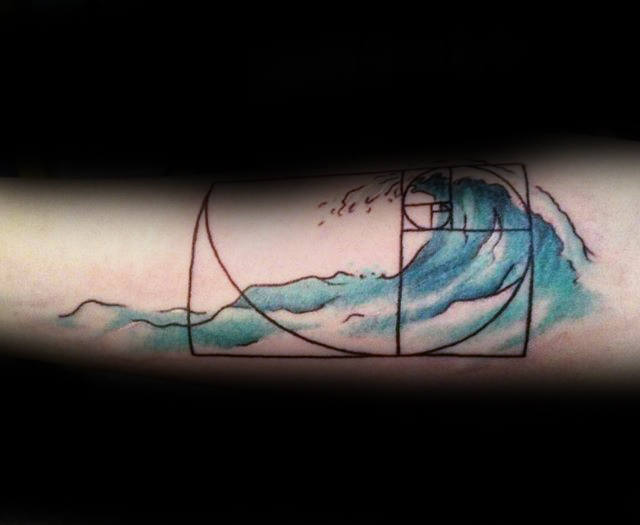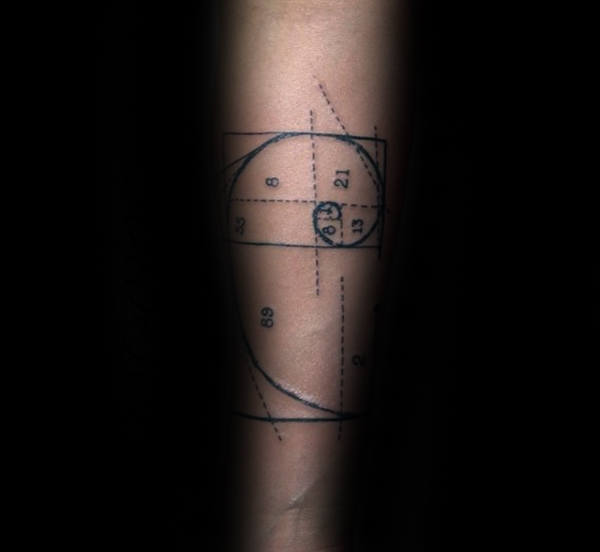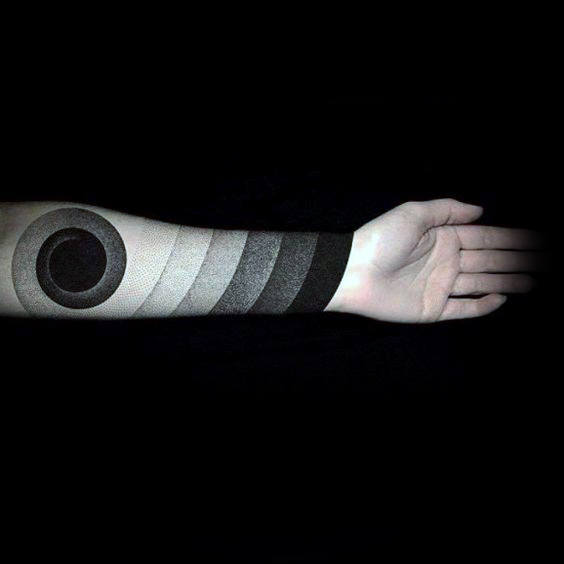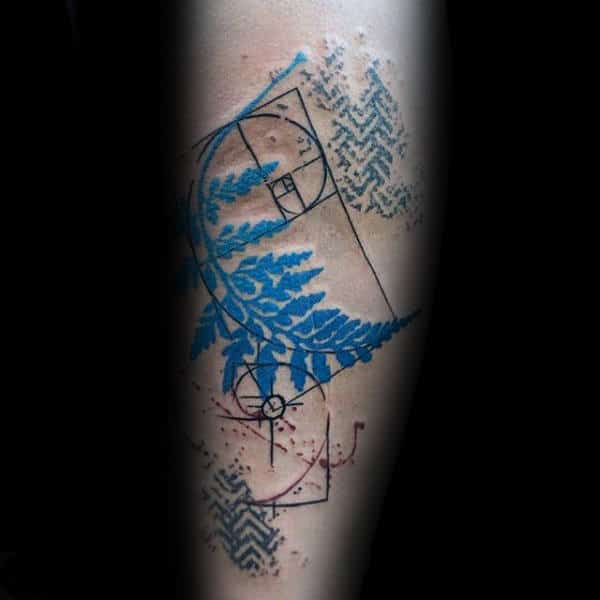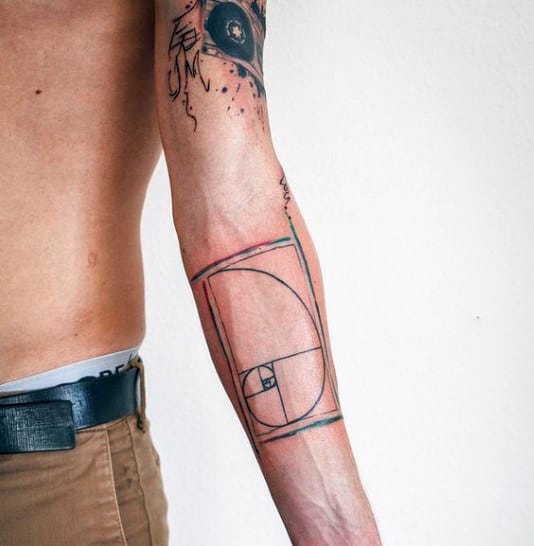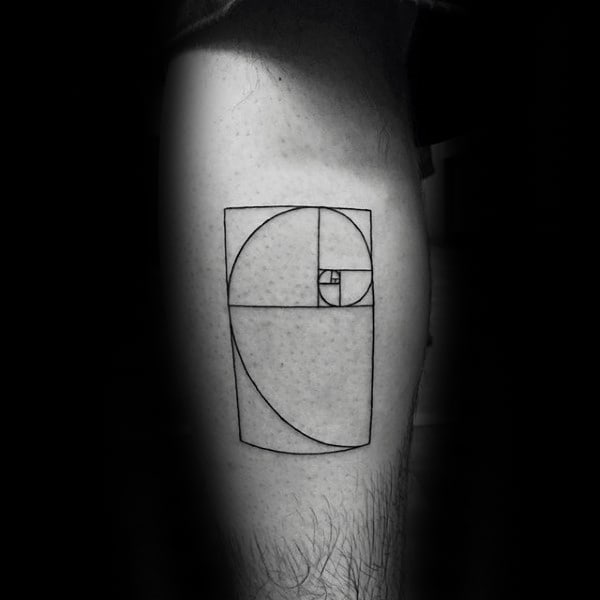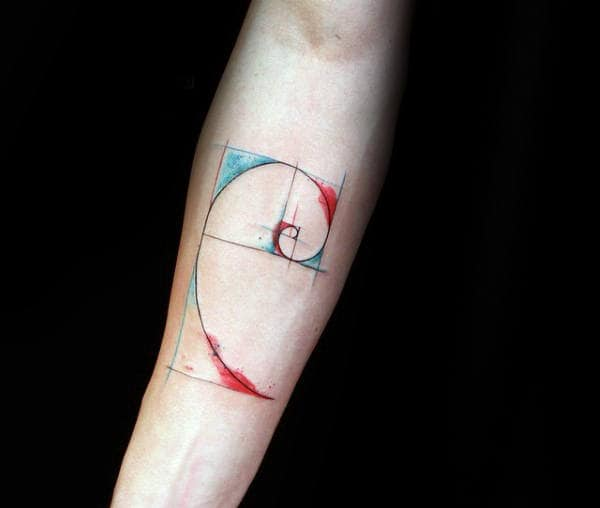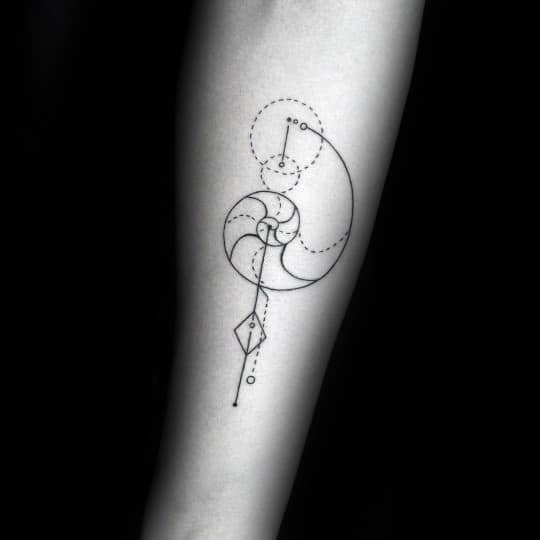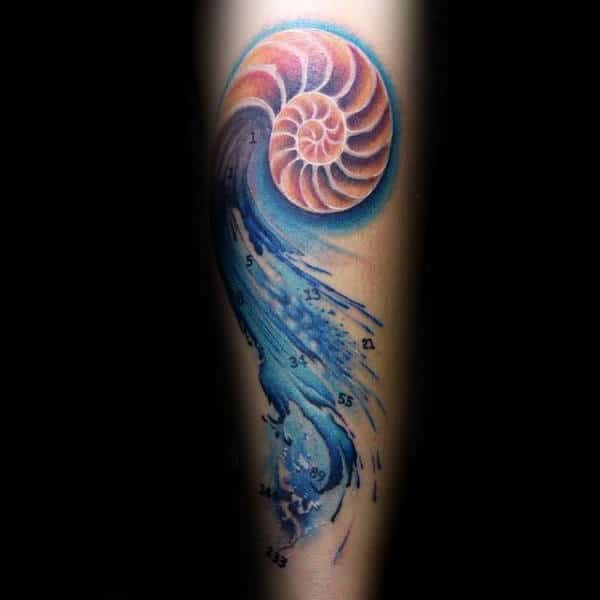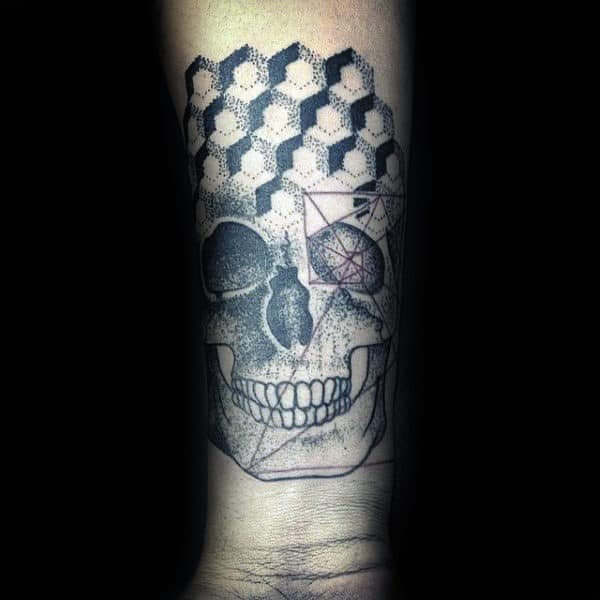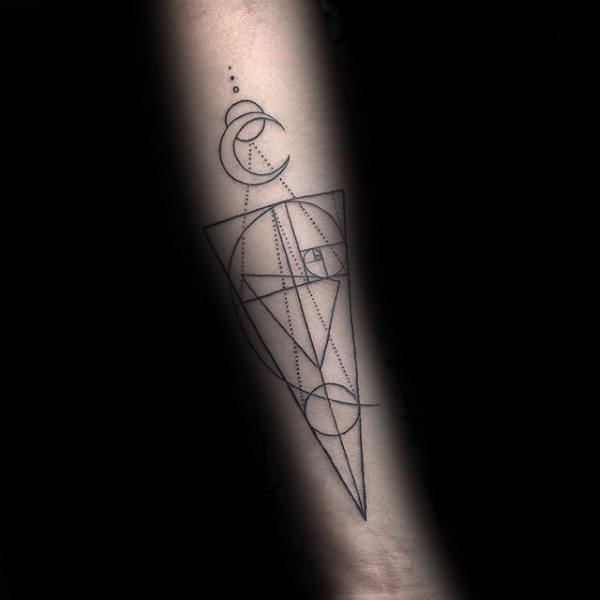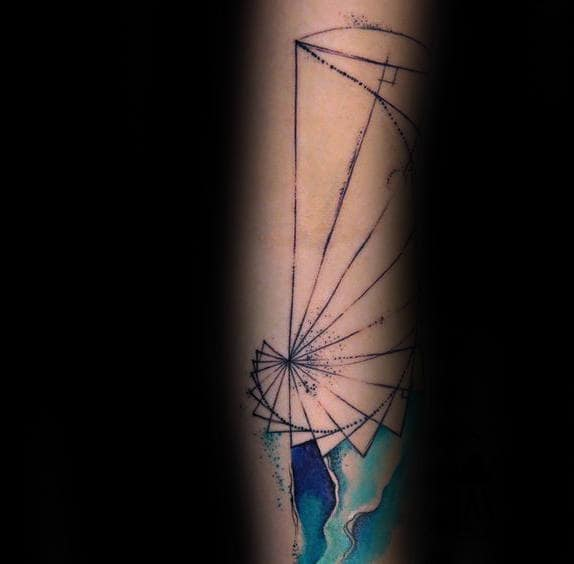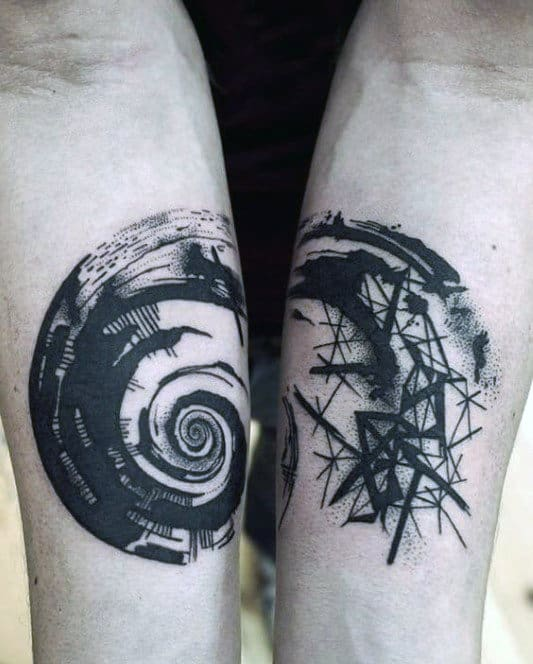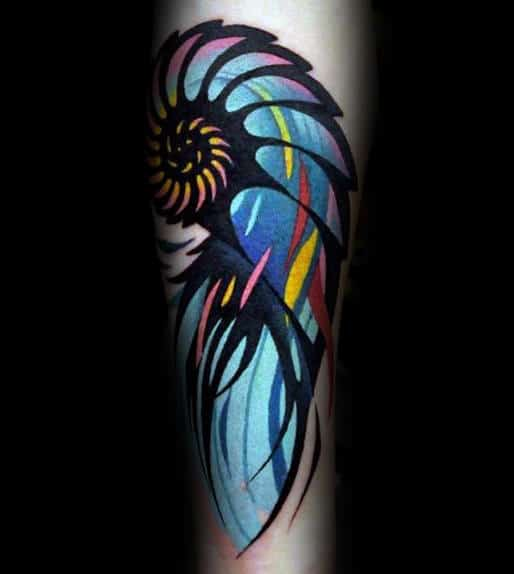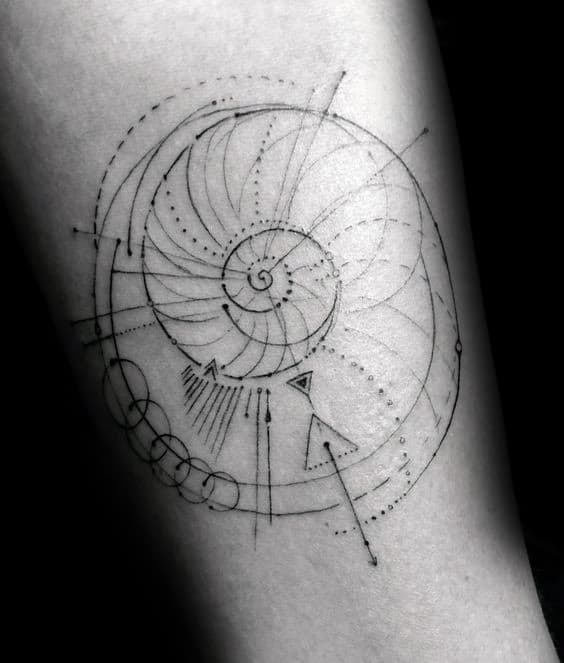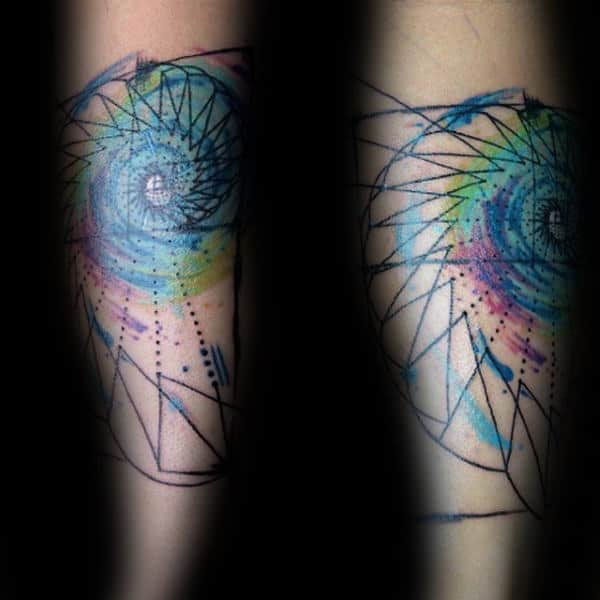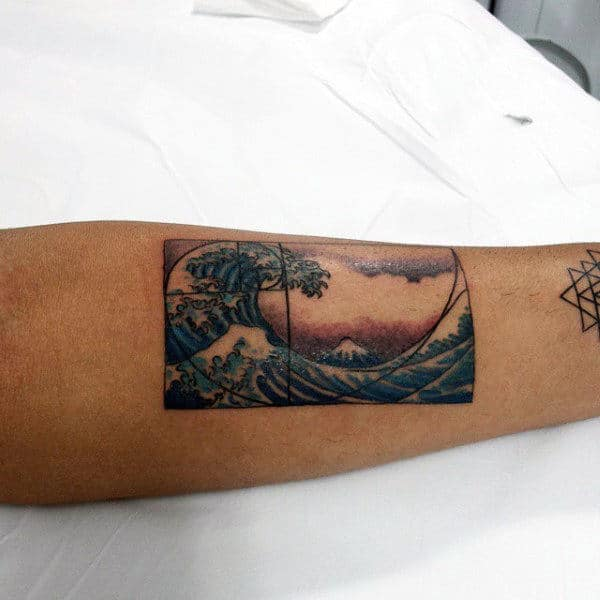## 2. Bicep Fibonacci Tattoos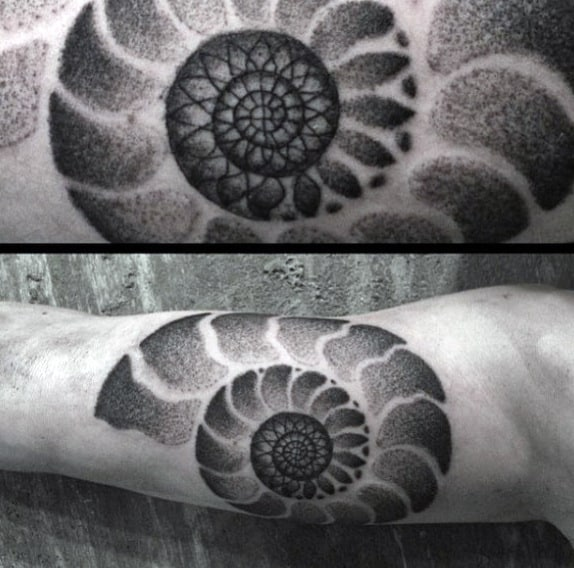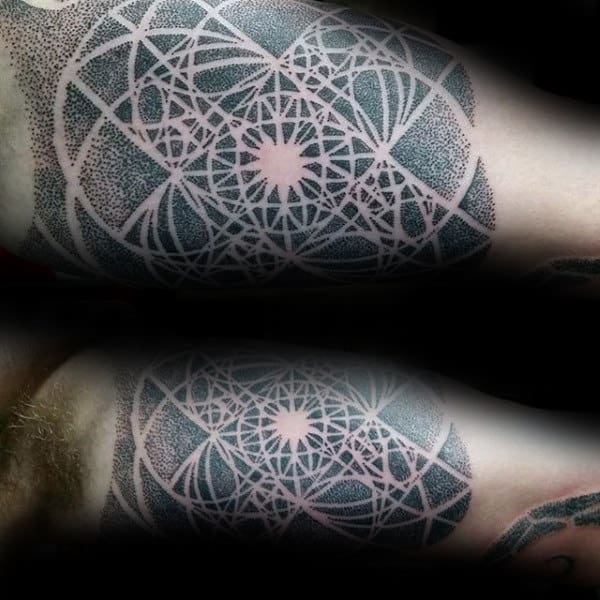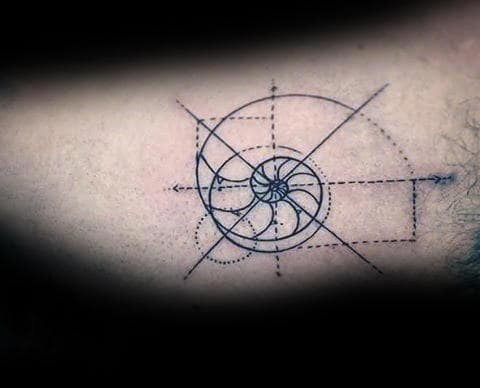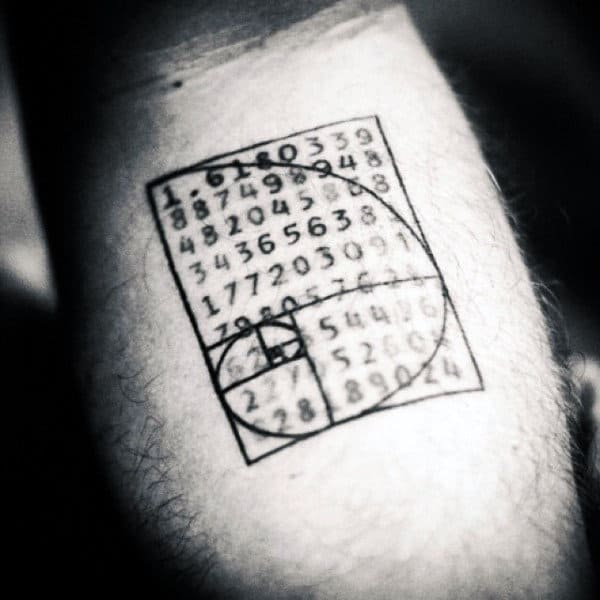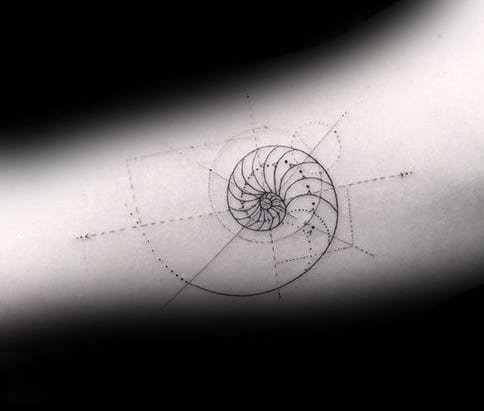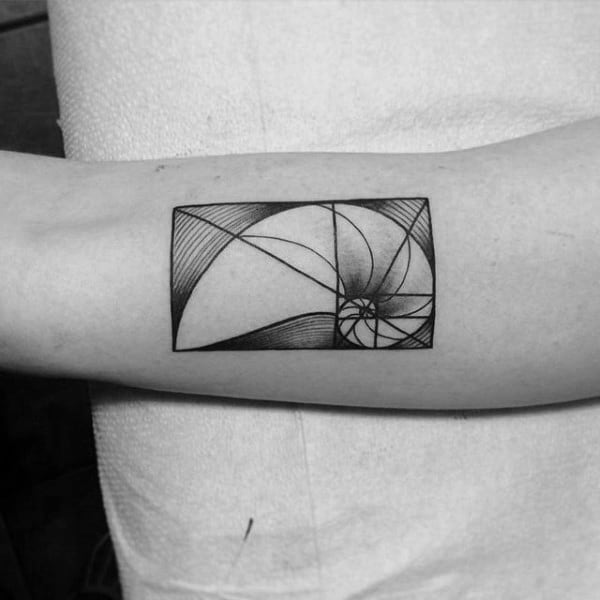## 3. Arm Fibonacci Tattoos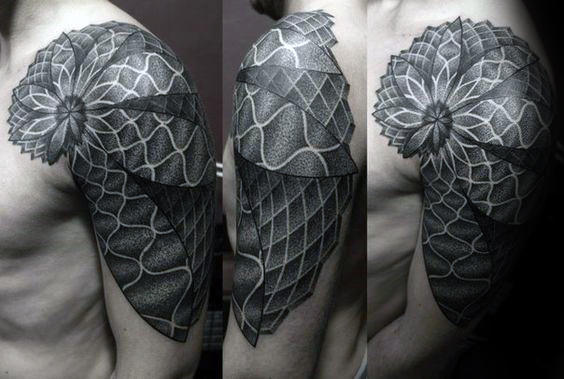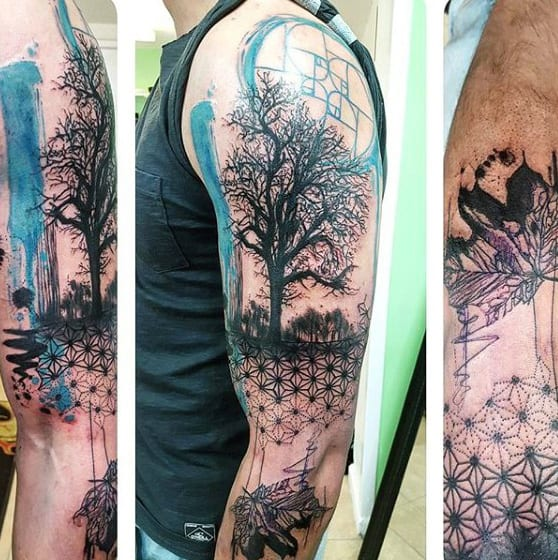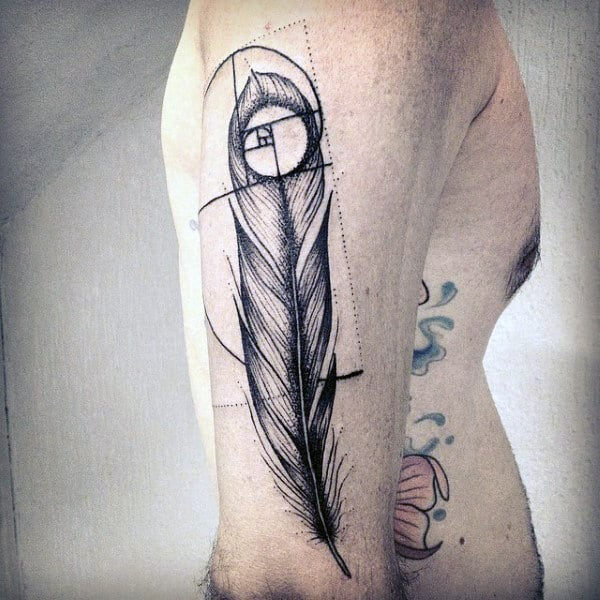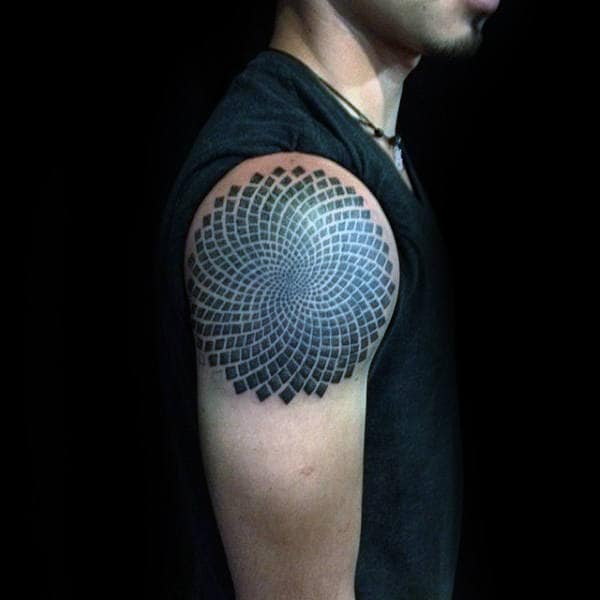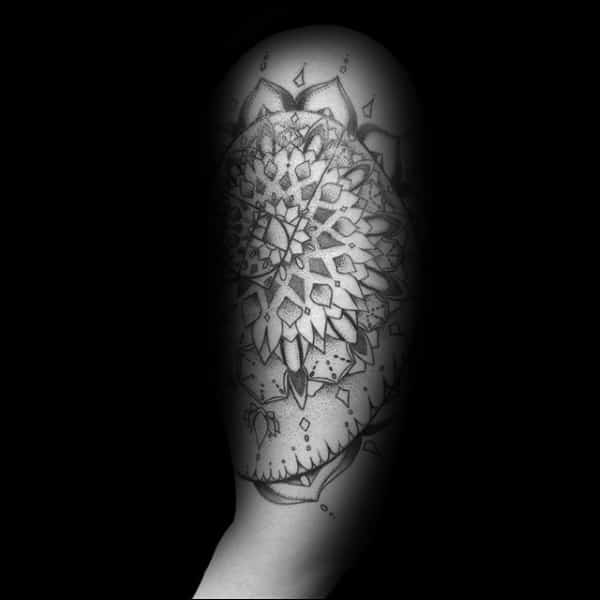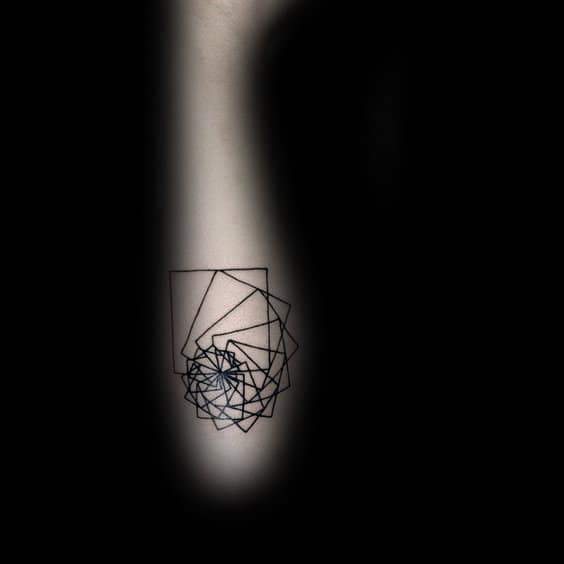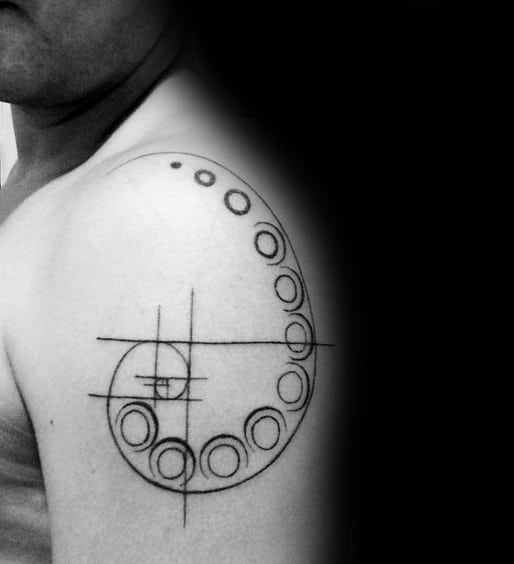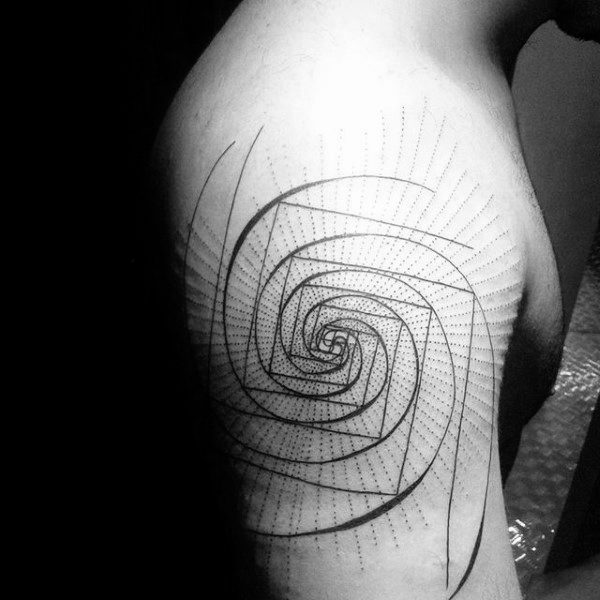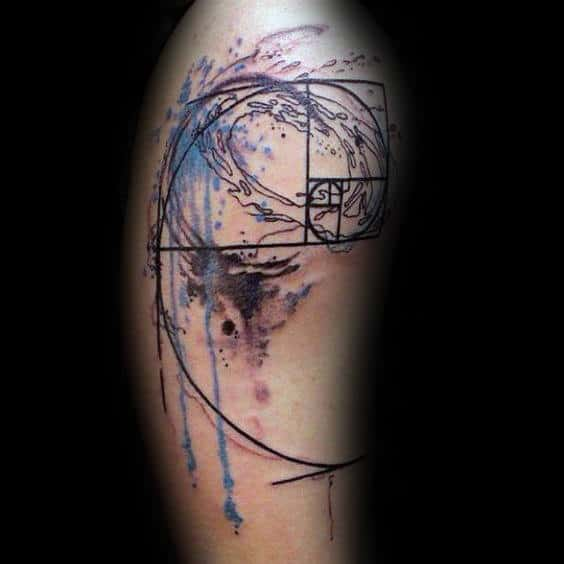## 4. Wrist Fibonacci Tattoos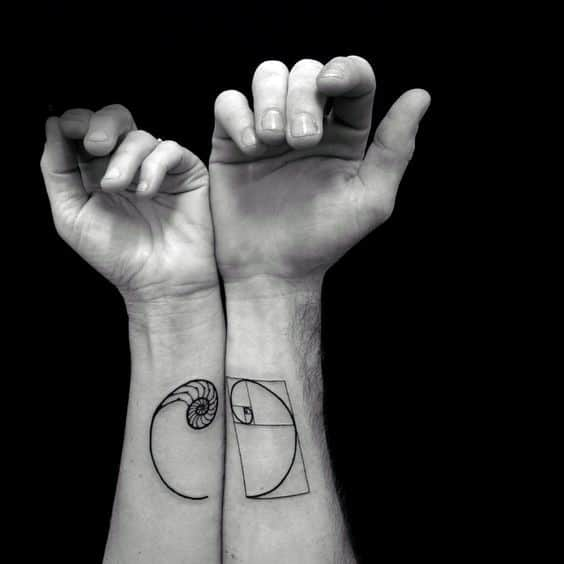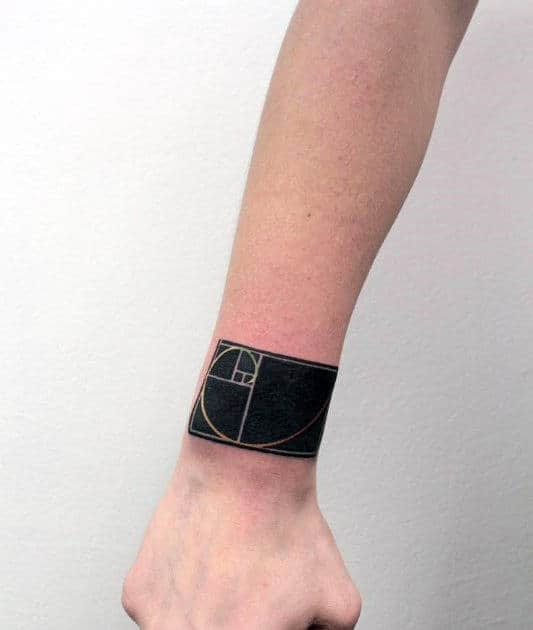## 5. Chest Fibonacci Tattoos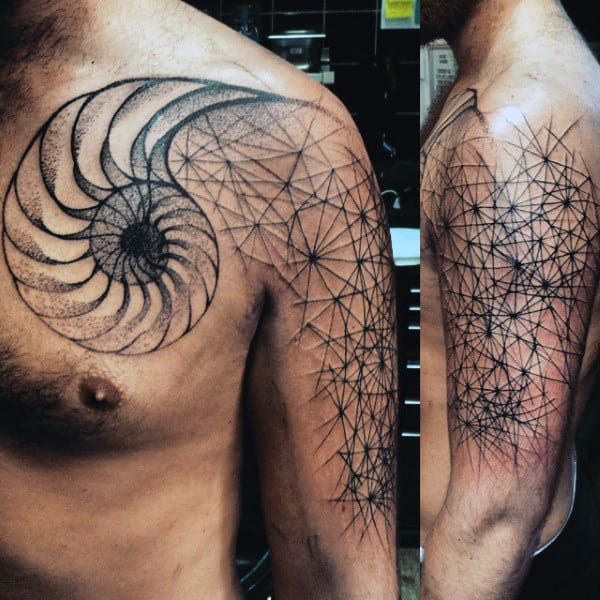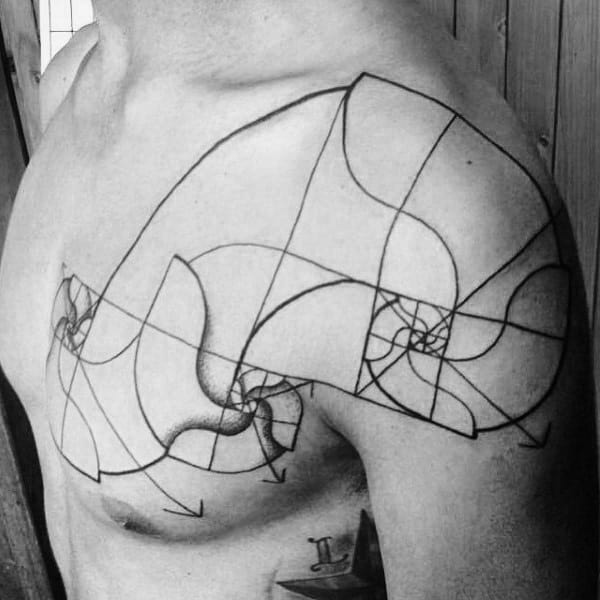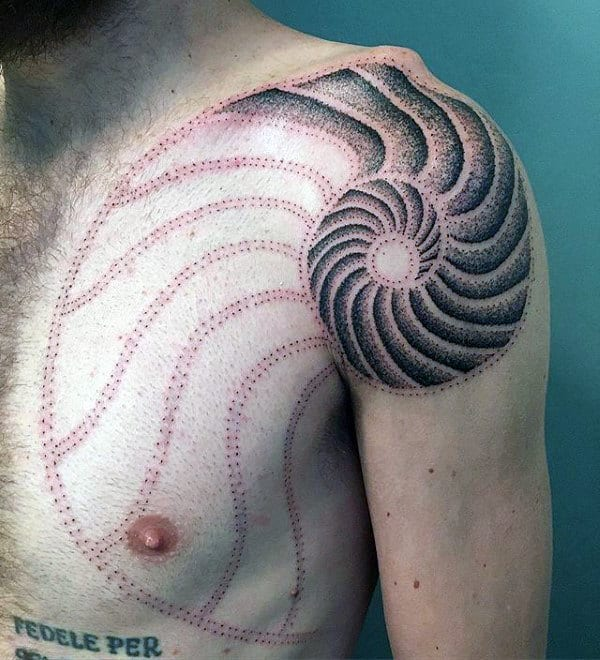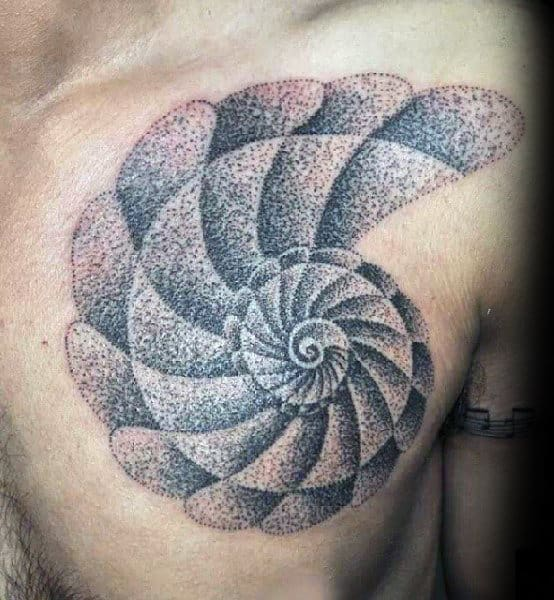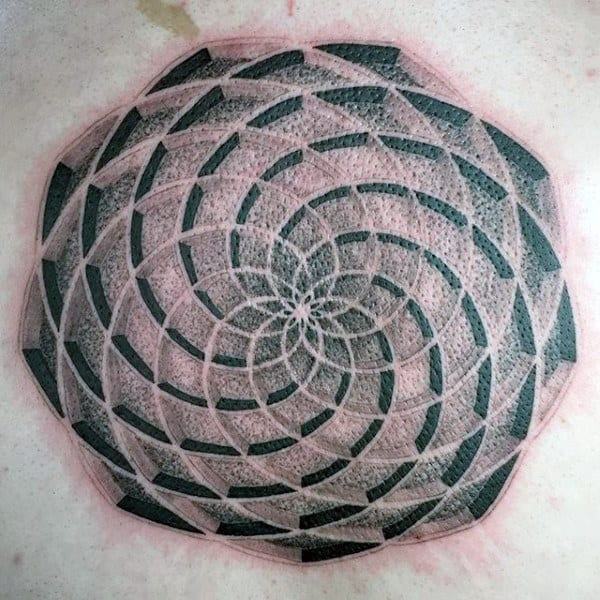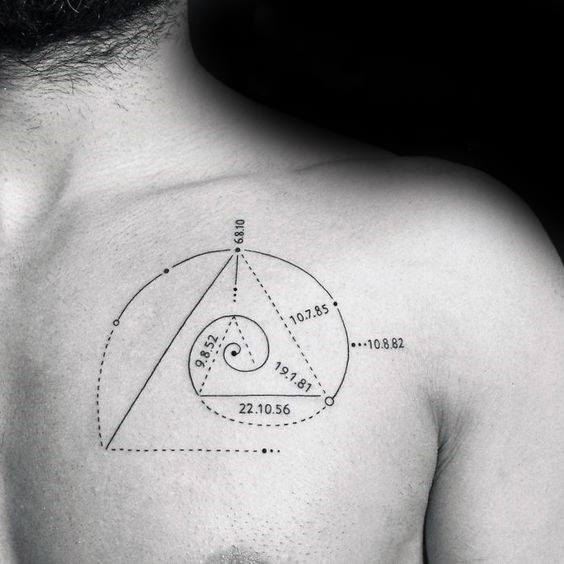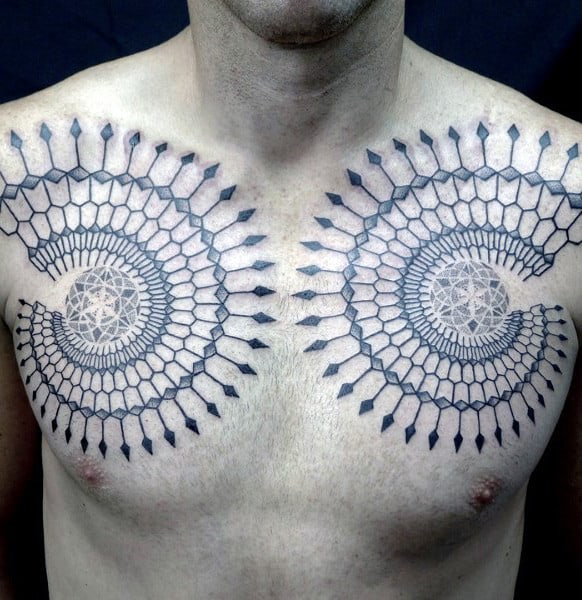## 6. Back Fibonacci Tattoos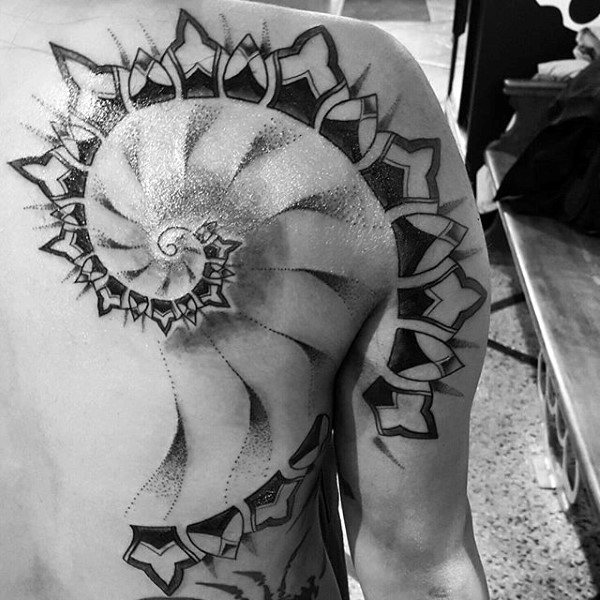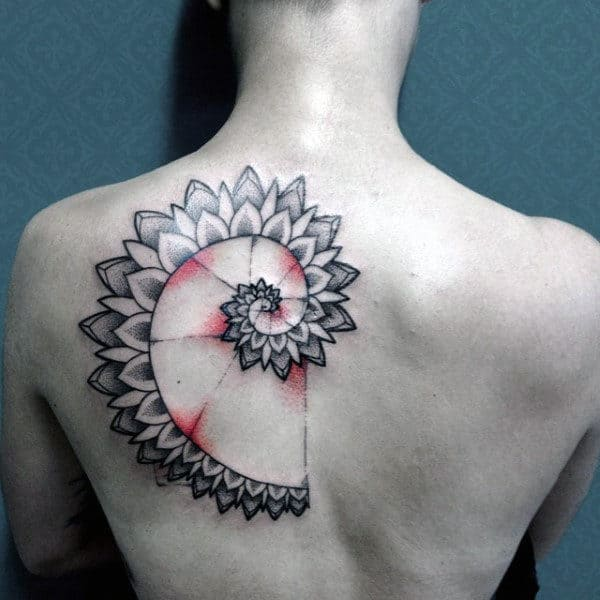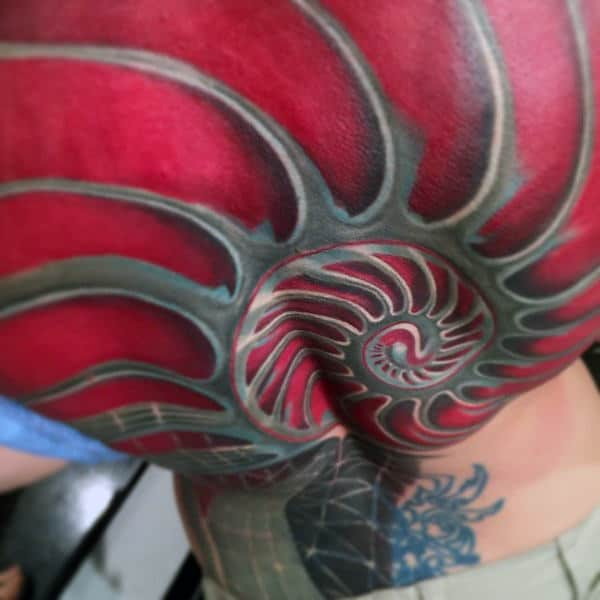## 7. Side Fibonacci Tattoos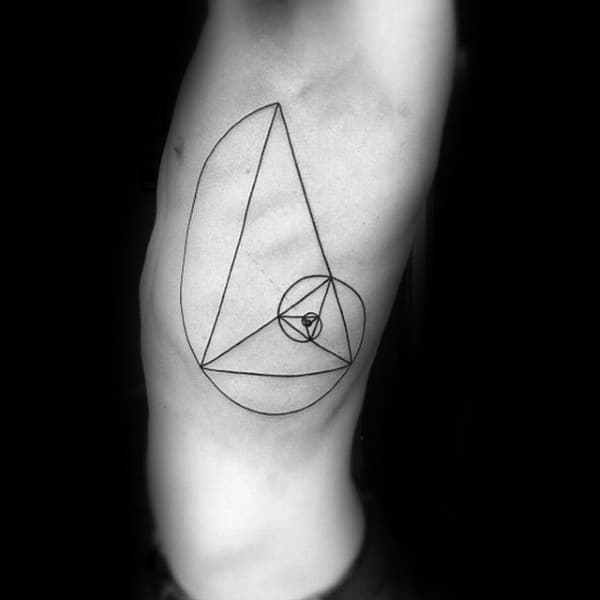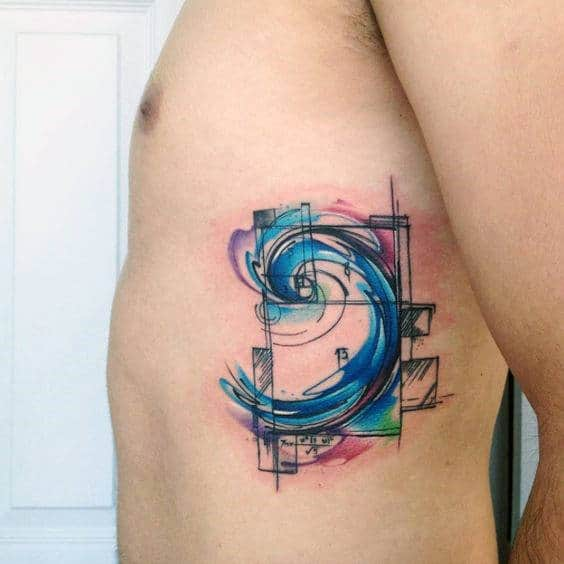## 8. Shoulder Fibonacci Tattoos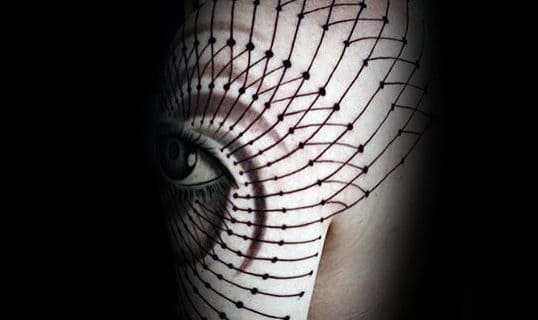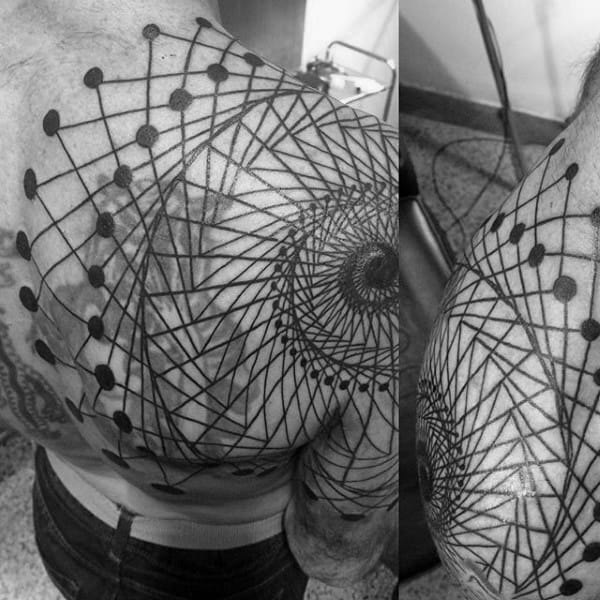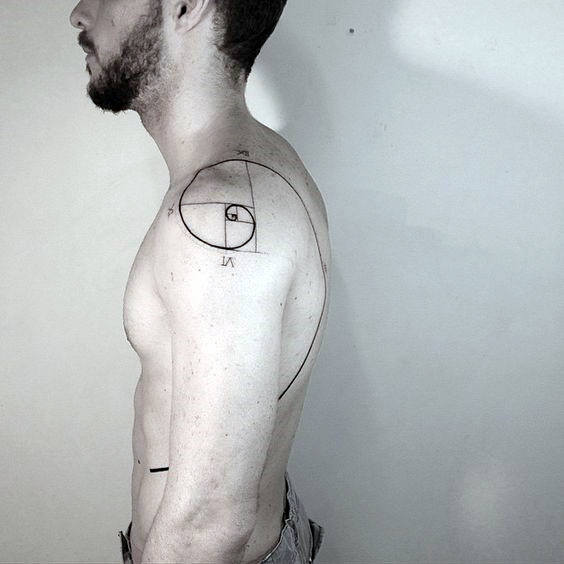## 9. Calf Fibonacci Tattoos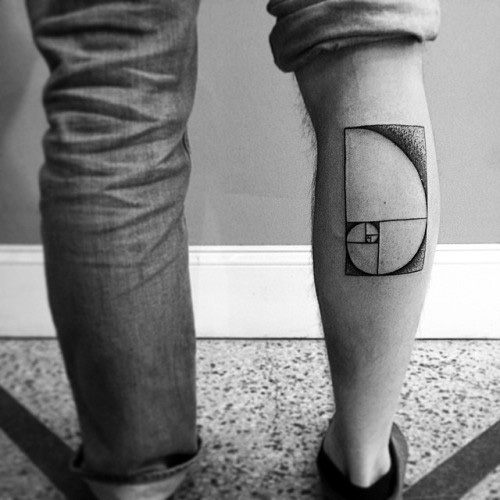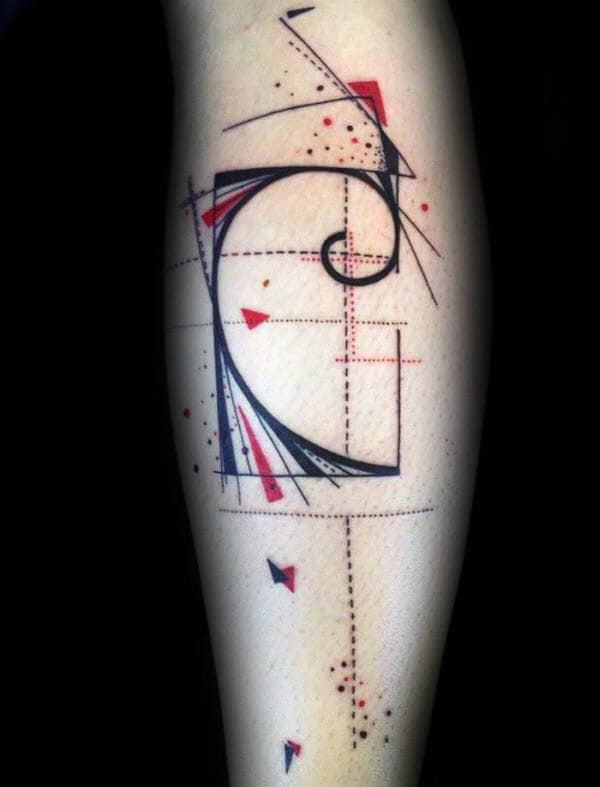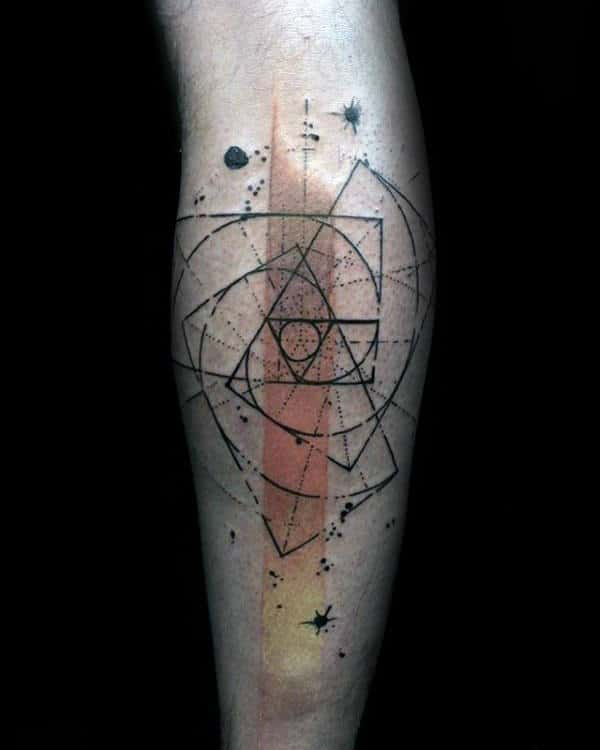## 10. Leg Fibonacci Tattoos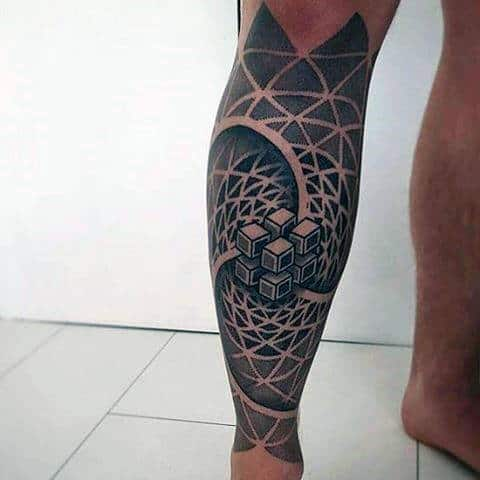## 11. Stomach Fibonacci Tattoos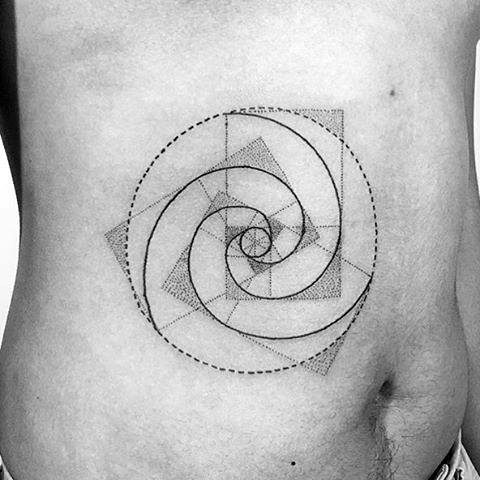## 12. Geometric Fibonacci Tattoos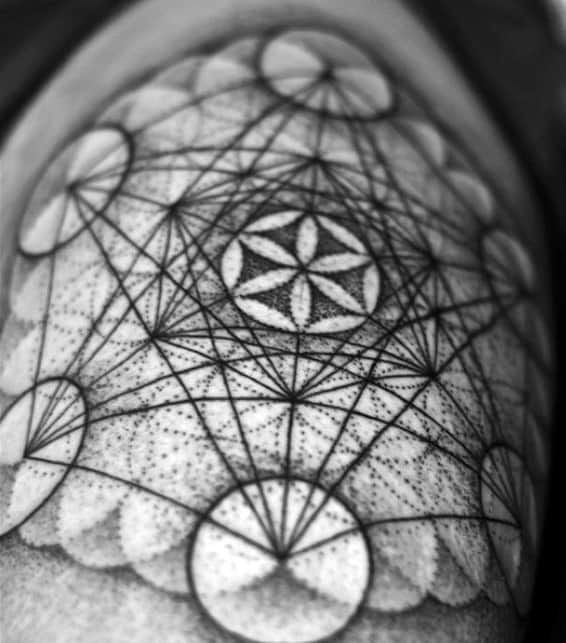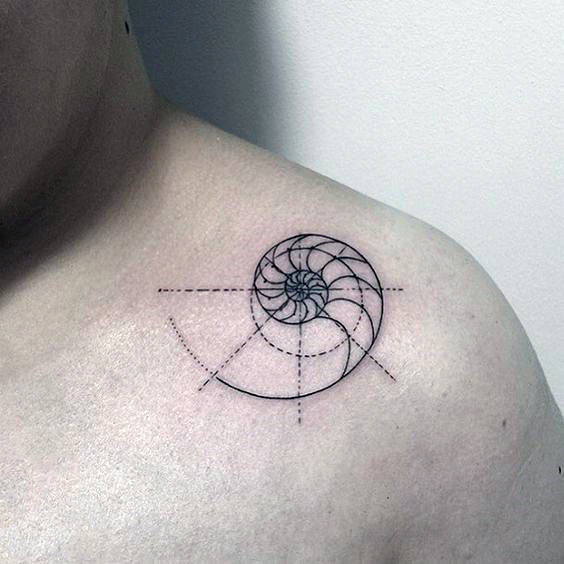## 13. Watercolor Fibonacci Tattoos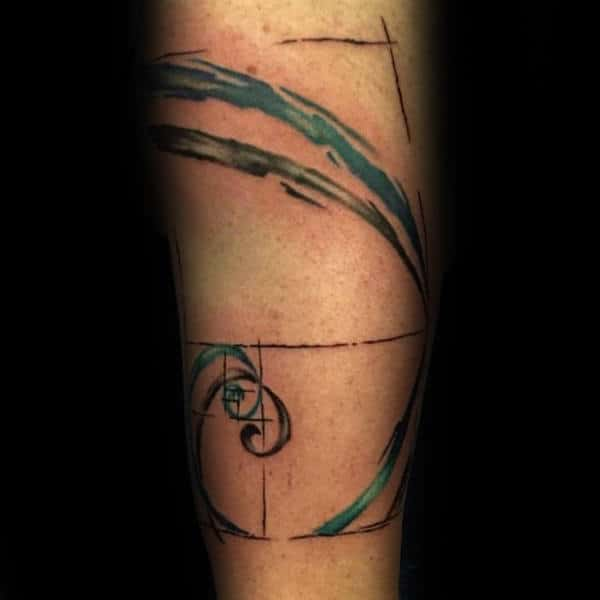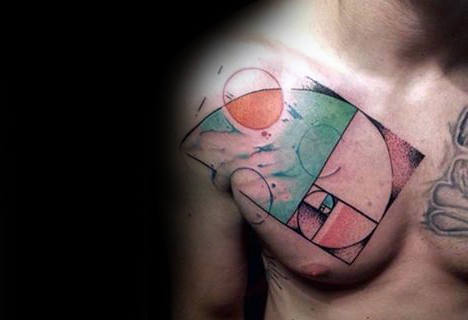## 14. Dotwork Fibonacci Tattoos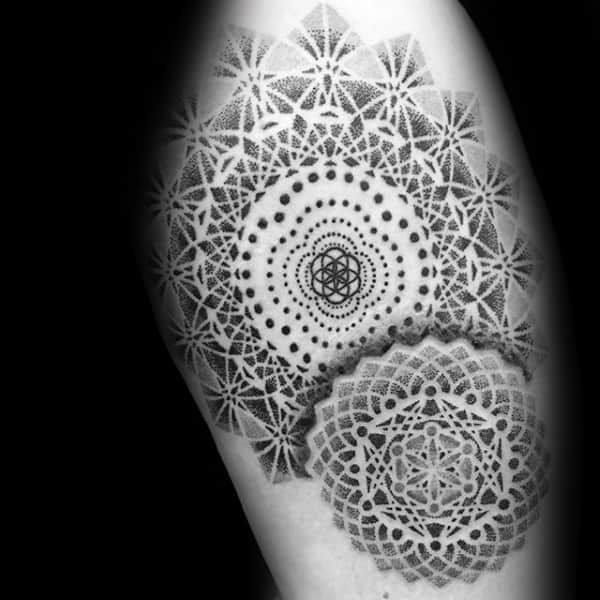Be A Gentleman